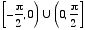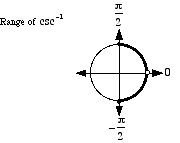index: click on a letter A B C D E F G H I J K L M N O P Q R S T U V W X Y Z A to Z index index: subject areas numbers & symbols sets, logic, proofs geometry algebra trigonometry advanced algebra & pre-calculus calculus advanced topics probability & statistics real world applications multimedia entrieswww.mathwords.com about mathwords website feedback

Inverse Cosecant

 csc-1 cosec-1 Csc-1 Cosec-1 arccsc arccosec Arccsc Arccosec

The inverse function of cosecant.

Basic idea: In order to find csc-1 2, we ask "what angle has cosecant equal to 2?" The answer is 30°. As a result we say csc-1 2 = 30°. In radians this is csc-1 2 = π/6.

More: There are actually many angles that have cosecant equal to 2. We are really asking "what is the simplest, most basic angle that has cosecant equal to 2?" As before, the answer is 30°. Thus csc-1 2 = 30° or csc-1 2 = π/6.

Details: What is csc-1 (–2)? Do we choose 210°, –30°, 330°, or some other angle? The answer is –30°. With inverse cosecant, we select the angle on the right half of the unit circle having measure as close to zero as possible. Thus csc-1 (–2) = –30° or csc-1 (–2) = –π/6.

In other words, the range of csc-1 is restricted to [–90°, 0) U (0, 90°] or. Note: csc 0 is undefined, so 0 is not in the range of csc-1.

Note: arccsc refers to "arc cosecant", or the radian measure of the arc on a circle corresponding to a given value of cosecant.

Technical note: Since none of the six trig functions sine, cosine, tangent, cosecant, secant, and cotangent are one-to-one, their inverses are not functions. Each trig function can have its domain restricted, however, in order to make its inverse a function. Some mathematicians write these restricted trig functions and their inverses with an initial capital letter (e.g. Csc or Csc-1). However, most mathematicians do not follow this practice. This website does not distinguish between capitalized and uncapitalized trig functions.